Published: 28 November 2019

# Optimization and analysis of novel thermoelectric module

Deepali Atheaya2
1Engineering Physics Department, School of Engineering and Applied Sciences, Bennett University, Tech Zone – II, Greater Noida, 201310, UP, India
2Mechanical and Aerospace Engineering Department, School of Engineering and Applied Sciences, Bennett University, Tech Zone – II, Greater Noida, 201310, UP, India
Corresponding Author:
Views 362

#### Abstract

Optimization and analysis of novel thermoelectric module is proposed in this research paper. The simulation for four thermoelectric modules were performed in COMSOL Multiphysics 5.4 software and detailed analysis of these thermoelectric modules were carried out. The three thermoelectric modules showed the efficiency and power output above-average thermoelectric modules. It also indicated that lead telluride based thermoelectric modules could be used in isolated areas due to toxicity of lead whereas tetrahedrite based thermoelectric module could be used in non-isolated areas due to its non-toxic properties. The proposed thermoelectric modules can be utilized in applications such as industries, deep space explorations, automobiles, thermal power plants, renewable electricity generation, hybrid renewable systems, etc. in an economically viable manner.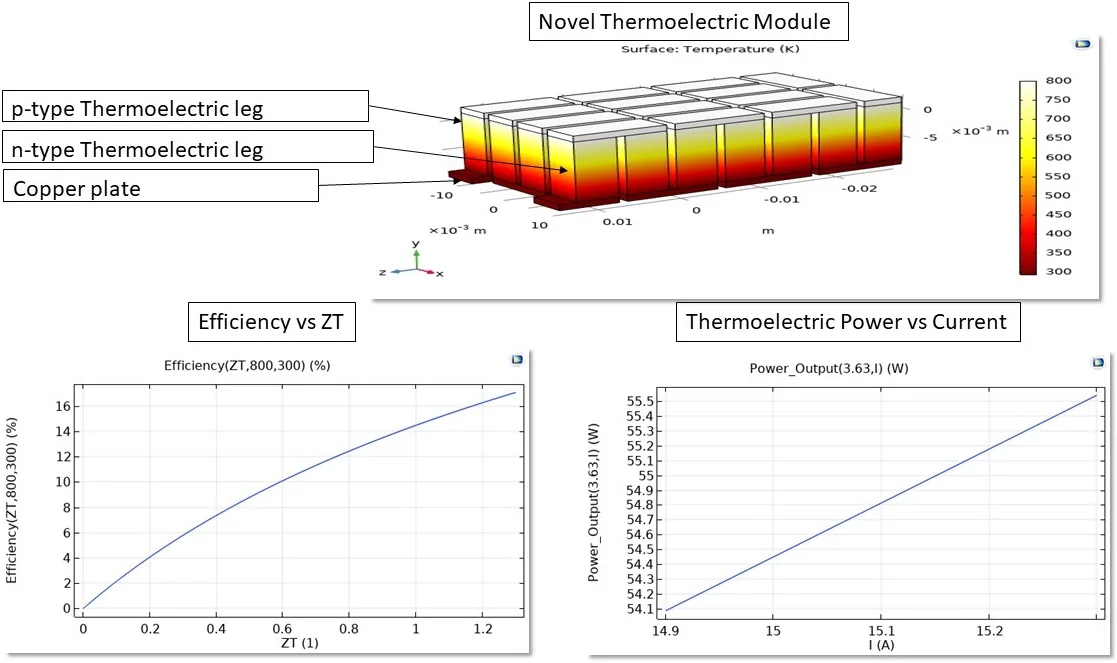#### Highlights

• Optimization and analysis of novel thermoelectric module is proposed in this research paper.
• The simulation for four thermoelectric modules were performed in COMSOL Multiphysics 5.4 software and detailed analysis of these thermoelectric modules were carried out.
• The three thermoelectric modules showed the efficiency and power output above-average thermoelectric modules.
• The proposed thermoelectric modules can be utilized in applications such as, automobiles, thermal power plants, renewable electricity generation, hybrid systems, etc. in an economically viable manner.

## 1. Introduction

Thermoelectricity works on Seebeck principle which states, “The Seebeck effect is a phenomenon in which the temperature difference between two dissimilar electrical conductors or semiconductors produces voltage difference between the two substances”. The work to increase performance efficiency ($\eta$) of thermoelectric modules is going since decades and so far 5-8 % efficiency ($\eta$) could be achieved . In 2009, Alphabet Energy invested \$49.5 million to develop waste heat recovery thermoelectric generator and claimed to have an efficiency ($\eta$) of 5 % and ensured to go above 10 % in upcoming years. A group of researchers from Swiss Federal Laboratories for Materials Testing and Research, High Voltage Laboratories and Institute of Physics of ASCR experimented GdCo0.95Ni0.05O3 and CaMn0.98Nb0.02O3 thermoelectric materials and thermoelectric modules and evaluated their power characteristics . Fu et al.  designed quaternary alloys of Pb1-xMgxTe0.8Se0.2 and achieved a figure of merit ($zT$) of 2.2 at 820 K. Chen  from Queen Mary University of London provided a comprehensive study on synthesis method, crustal structures and thermoelectric properties of tetrahedrite compounds. Scientists from Panasonic experimented and studied the thermoelectric properties of skutterudites and achieved a figure of merit ($zT$) of 1.1 at 820 K for the composition CoSb2.875Te0.125 . Recently, Lan et al.  predicted fuel economy potential for a skutterudite thermoelectric generator and observed that 25 %-50 % of fuel efficiency is obtained in light-duty vehicles.

The simulation of various combinations of thermoelectric couples were performed and it was noted that Pb1-xMgxTe0.8Se0.2 and $n$-type PbTe, Pb1-xMgxTe0.8Se0.2 and CoSb3-xTex, Pb1-xMgxTe0.8Se0.2 and CaMn0.98Nb0.02O3, Cu12Sb4S13 and CoSb3-xTex indicated higher efficiency ($\eta$) than other thermoelectric couples . In this research paper, performance analysis of these optimized novel thermoelectric modules was carried out.

## 2. Analysis of thermoelectric module

Table 1 shows the simulated thermoelectric modules and their calculated and validated properties. From the table it can be concluded, that Pb1-xMgxTe0.8Se0.2 and $n$-type PbTe, Pb1-xMgxTe0.8Se0.2 and CoSb3-xTex, Cu12Sb4S13 and CoSb3-xTex showsefficiency ($\eta$) as predicted by Mane and Atheaya . The detailed analysis of Pb1-xMgxTe0.8Se0.2 and CaMn0.98Nb0.02O3 showed efficiency ($\eta$) below 5 %. Therefore Pb1-xMgxTe0.8Se0.2 and CaMn0.98Nb0.02O3 cannot be used to develop an efficient thermoelectric module.

Table 1Thermoelectric modules and their properties

 Thermoelectric materials ZT $\eta$(%) Power output(W) Voltage(V) Current(A) P-type material N-type material Pb1-xMgxTe0.8Se0.2 $n$-type PbTe 1.30 17 54.92 3.63 15.13 Pb1-xMgxTe0.8Se0.2 CoSb3-xTex 1.20 16 60.96 3.20 19.05 Cu12Sb4S13 CoSb3-xTex 0.6 10 14.57 2.77 5.26 Pb1-xMgxTe0.8Se0.2 CaMn0.98Nb0.02O3 0.11 2.4 6.31 3.41 1.85

The unitless figure of merit ($ZT$) of thermoelectric module is the key entity to measure thermoelectric efficiency ($\eta$) and is formulated as follows :

1
$ZT=\frac{\left({{S}_{p}-{S}_{n}\right)}^{2}T}{{\left(\sqrt{{\rho }_{n}{k}_{n}}+\sqrt{{\rho }_{p}{k}_{p}}\right)}^{2}}.$

The thermoelectric performance efficiency ($\eta$) is mainly dependent on the figure of merit ($ZT$) and is formulated as follows :

2
$\eta =\frac{{T}_{H}-{T}_{C}}{{T}_{H}}\frac{\sqrt{\left(1+ZT\right)}-1}{\sqrt{\left(1+ZT\right)}+\frac{{T}_{C}}{{T}_{H}}}.$

Thermoelectric voltage ($V$) is induced when a temperature gradient is created and is formulated as follows :

3
$V=\left({S}_{p}-{S}_{n}\right)T.$

Thermoelectric current ($I$) was calculated by using Ohms law. The net resistance ($R$) of the thermoelectric module was calculated by summing the resistance of thermoelectric legs:

4
${R}_{p}=12{\rho }_{p}\frac{l}{A},{R}_{n}=12{\rho }_{n}\frac{l}{A},R={R}_{p}+{R}_{n},I=\frac{V}{R}.$

Thermoelectric power output ($P$) is simply the product of thermoelectric voltage ($V$) and the thermoelectric current ($I$):

5
$P=VI.$

## 3. Results and discussion

All thermoelectric modules were simulated and detailed investigation was carried out in COMSOL Multiphysics 5.4 software. The properties of thermoelectric materials were also validated in the simulation. The highest efficiency was found to be 17 % and the results of this thermoelectric module have been discussed further.

The thermoelectric module was designed in COMSOL Multiphysics. The boundary conditions set to the module were 800 K and 300 K at the upper and lower surface respectively. Tetrahedral elements meshing was set manually for thermoelectric legs as well as copper metallic plates. Extremely fine mesh with minimum element size 8.6×10-6 meters and maximum element size 5.6×10-4 meters were set for thermoelectric legs to provide accurate results. Extra fine mesh with minimum element size 6.4×10-5 meters and maximum element size 1.5×10-3 meters were set for copper plates to reduce the computational time. High level element quality optimization was set to perform efficient meshing.

Fig. 1Temperature distribution along the surface of the thermoelectric module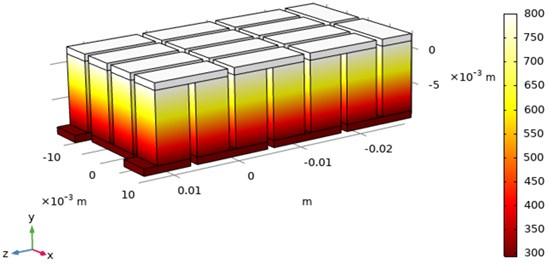Fig. 1 displays the temperature distribution along the surface of the thermoelectric module. The module has a dimension of 43 mm×23 mm×12 mm and it comprises of twenty-four thermoelectric legs which were attached to copper metallic plates. The temperature at the hot terminal was set at 800 K and the temperature at the cold terminal was set at 300 K. Fig. 1 displays that the temperature is evenly distributed along the surface of the thermoelectric module.

Fig. 2Figure of merit (ZT) with respect to temperature difference (T) for Pb1-xMgxTe0.8Se0.2 and n-type PbTe thermoelectric module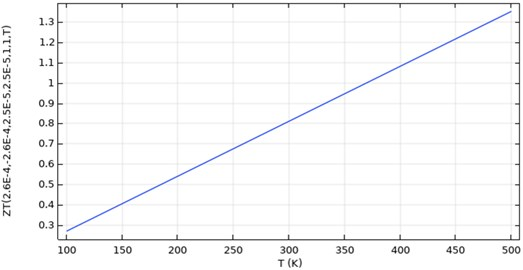Fig. 2 demonstrates the figure of merit $\text{(}ZT\text{)}$ for Pb1-xMgxTe0.8Se0.2 and $n$-type PbTe thermoelectric module for various temperature difference ($T$). At temperature difference of 100 K it was observed that figure of merit ($ZT$) of approximately 0.3 was achieved whereas, at a temperature difference of 500 K, the figure of merit ($ZT$) of approximately 1.3 was achieved.

Fig. 3Efficiency (η) with respect to figure of merit (ZT) for Pb1-xMgxTe0.8Se0.2 and n-type PbTe thermoelectric module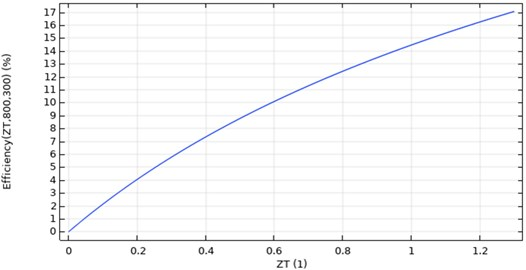The efficiency ($\eta$) for Pb1-xMgxTe0.8Se0.2 and $n$-type PbTe thermoelectric module has been presented in Fig. 3. It was observed that for figure of merit ($ZT$) of 1.3, an efficiency ($\eta$) of 17 % was achieved. Consequently, Pb1-xMgxTe0.8Se0.2 and $n$-type PbTe thermoelectric module had the highest thermoelectric performance efficiency ($\eta$) and it may be utilized to fabricate an efficient thermoelectric generator.

Fig. 4Thermoelectric power output (P) with respect to thermoelectric current (I) for Pb1-xMgxTe0.8Se0.2 and n-type PbTe thermoelectric module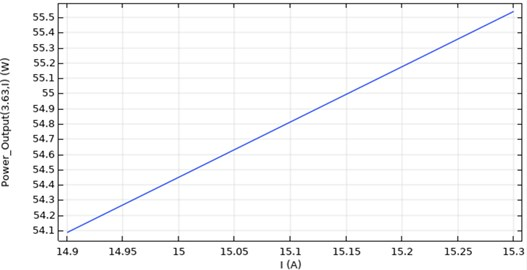Fig. 4 displays the thermoelectric power output ($P$) with respect to thermoelectric current ($I$) for Pb1-xMgxTe0.8Se0.2 and $n$-type PbTe thermoelectric module. It was observed that for thermoelectric current ($I$) of 15.13 A, power output ($P$) of 54.92 W was achieved. Further, the current can be increased by increasing the area-length ratio of the thermoelectric module or by decreasing resistivity of the thermoelectric module.

Fig. 5Thermoelectric voltage output (V) for Pb1-xMgxTe0.8Se0.2 and n-type PbTe thermoelectric module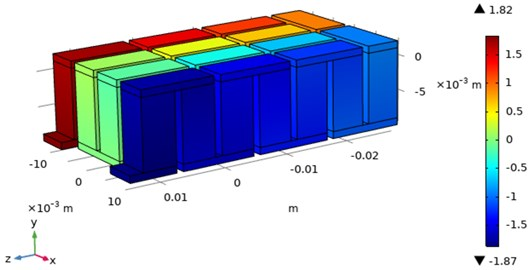Fig. 5 displays the thermoelectric voltage output ($V$) for Pb1-xMgxTe0.8Se0.2 and $n$-type PbTe thermoelectric module. This analysis was carried out in multislice plot and volumetric plot and the mean value was considered as the thermoelectric voltage output ($V$). The multislice plot displayed 3.56 V whereas volumetric plot displayed 3.69 V. Therefore, thermoelectric voltage ($V$) of 3.63 V was considered to perform further analysis.

## 4. Conclusions

Based on present studies following conclusions have been made.

1) Pb1-xMgxTe0.8Se0.2 and $n$-type PbTe, Pb1-xMgxTe0.8Se0.2 and CoSb3-xTex, Cu12Sb4S13 and CoSb3-xTex showedhigherefficiency, thus, could be used in making efficient thermoelectric generators.

2) Pb1-xMgxTe0.8Se0.2 and CaMn0.98Nb0.02O3 showed efficiency below 5 %, thus, could not be used in making an efficient thermoelectric generator.

3) Lead telluride based thermoelectric modules could be used in isolated areas due to the toxicity of lead.

4) Tetrahedrite based thermoelectric module could be used in non-isolated areas due to its non-toxic properties and higher cost-efficiency.

5) Pb1-xMgxTe0.8Se0.2 and CoSb3-xTex thermoelectric module showed maximum thermoelectric power output.